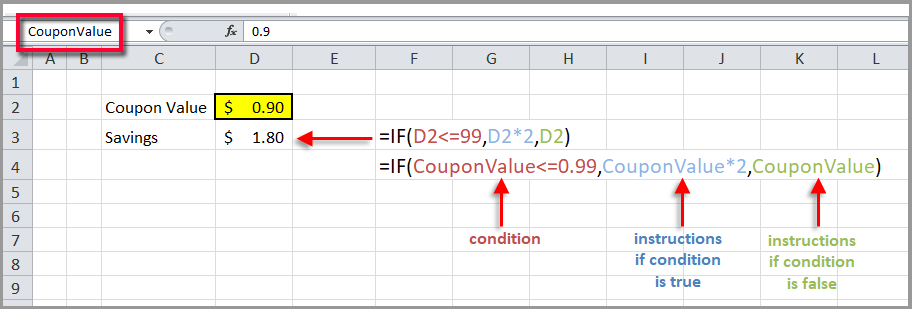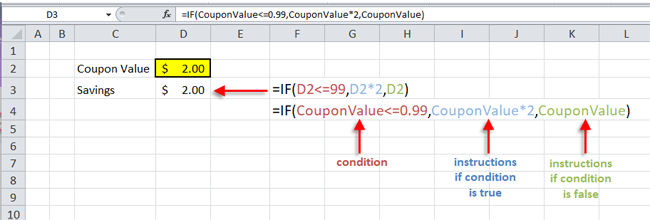Select Page

Share

In this case, you could use one of Excel’s most popular and useful functions, the IF function. You use an IF statement to ask Excel to test a condition and to return one value if the condition is met, and another value if the condition is not met.

Another way of saying it is that Excel makes a statement, and performs one calculation if the statement is true, and another calculation if the statement is false.

The format is like this:

=IF(condition, true instruction, false instruction)

For example, in English you would say “if the coupon is under 99 cents, multiply the coupon by 2, otherwise leave the coupon value as is.”  In this spreadsheet, the coupon value is entered in cell D2 and the formula is entered in cell D3.

If you place the coupon value in Cell D2, then you could substitute coupon in the statement above with D2.

To make it easier to audit you can also name cell D2 and call it CouponValue (no spaces in the range name). Then you can use that reference in the formula. To name the cell, you select it and type the name you want into the Name Box at the intersection of columns and rows in the top left.The formulas above are notated first with the cell value (D2) and second with the cell name (CouponValue). Otherwise, they are identical, but the second one is easier to read.

This is how it calculates when the CouponValue is higher than \$.99.Now every time you type a number into cell D2 the formula in cell D3 will recalculate, first checking to see if the coupon value is less than \$.99 or over \$.99.